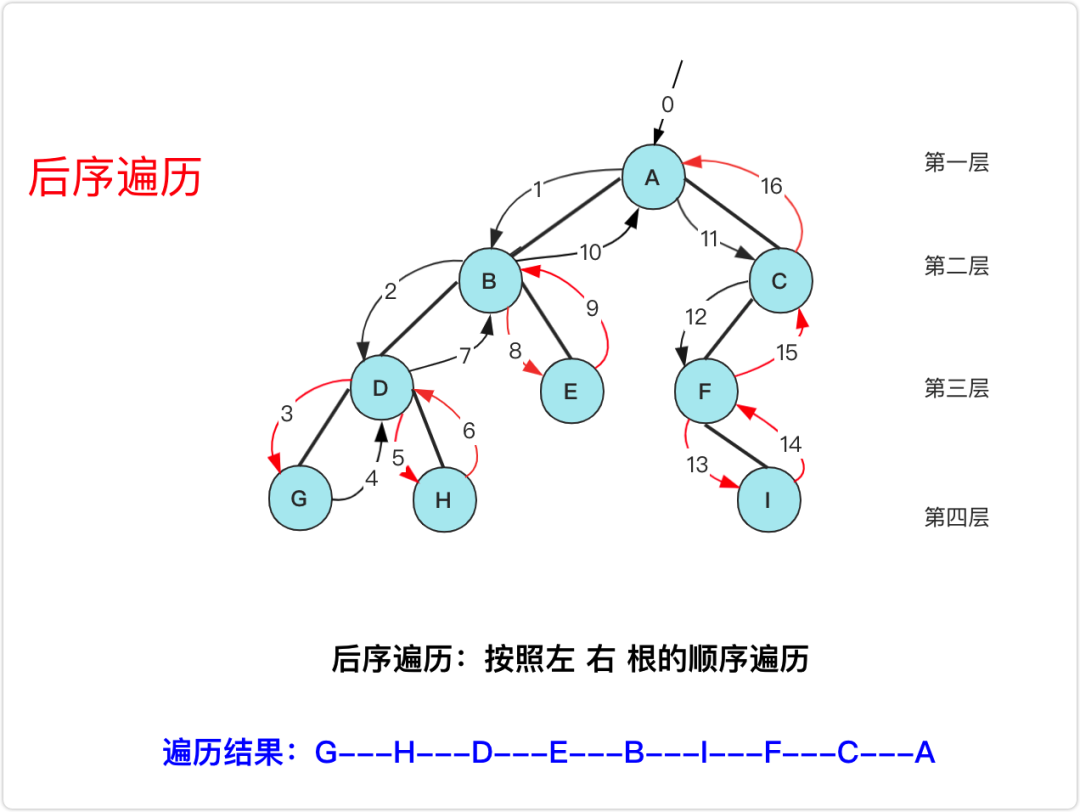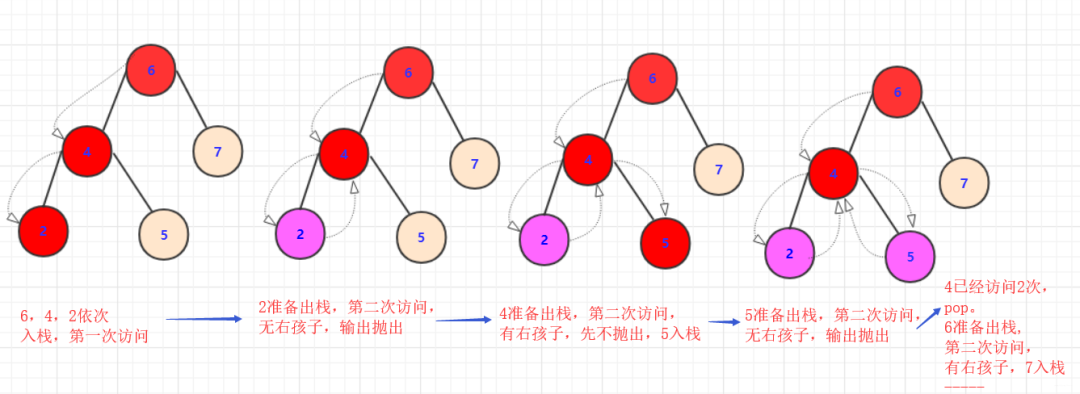# 二叉树各种遍历真的很难？大sai带你拿捏！（二）

joytrian

0收藏``````class Solution {
List<Integer>value=new ArrayList<>();
public List<Integer> postorderTraversal(TreeNode root) {
houxu(root);
return value;
}
private void houxu(TreeNode root) {
if(root==null)
return;
houxu(root.left);
houxu(root.right);//右子树回来
}
}````````````class Solution {
public List<Integer> postorderTraversal(TreeNode root) {
List<Integer> value=new ArrayList();
Stack<TreeNode> q1 = new Stack();
Map<TreeNode,Integer >map=new HashMap<>();
while(!q1.isEmpty()||root!=null)
{
if (root!=null) {
q1.push(root);
map.put(root, 1); //t.value标记这个值节点出现的次数
root=root.left;
}
else {
root=q1.peek();
if(map.get(root)==2) {//第二次访问，抛出
q1.pop();
root=null;//需要往上走
}
else {
map.put(root, 2);
root=root.right;
}
}
}
return value;
}
}``````

``````class Solution {
public List<Integer> postorderTraversal(TreeNode root) {
TreeNode temp=root;//枚举的临时节点
List<Integer>value=new ArrayList<>();
TreeNode pre=null;//前置节点
Stack<TreeNode>stack=new Stack<>();

while (!stack.isEmpty()||temp!=null){

while(temp!=null){
stack.push(temp);
temp=temp.left;
}
temp=stack.pop();
if(temp.right==pre||temp.right==null)//需要弹出
{
pre=temp;
temp=null;//需要重新从栈中抛出
}else{
stack.push(temp);
temp=temp.right;
}

}
return value;
}
}``````

``````class Solution {
public List<Integer> postorderTraversal(TreeNode root) {
List<Integer>value=new ArrayList();
Stack<TreeNode> q1 = new Stack();
while(!q1.isEmpty()||root!=null)
{
while (root!=null) {
q1.push(root);
root=root.right;
}
root=q1.pop();//抛出
root=root.left;//准备访问其右节点
}
Collections.reverse(value);//将结果翻转
return value;

}
}``````帖子
视频
声望
粉丝
社区精华内容